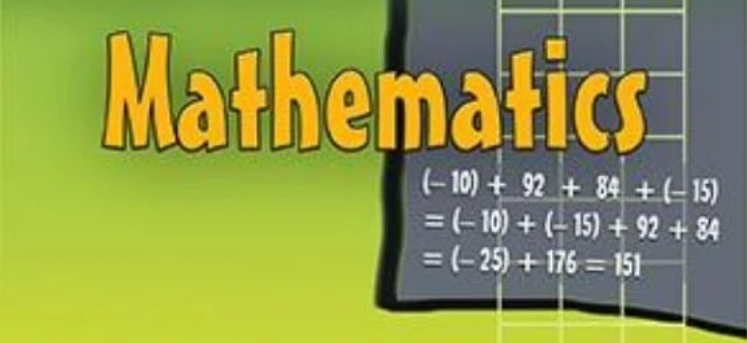## New NCERT Solutions for Class 6 Maths – Latest Solutions

NCERT Solutions for Class 6 Maths – Students can download NCERT Solutions for Class 6 Maths from this page. It is an important resource which can be used by the students preparing for Class 6th exam. The solutions provided here have been prepared in such a way that it is easy for the students to understand and is useful for them for scoring higher marks in the exam. Many students fear mathematics, however, it is one of the most interesting subject. In Class 6 NCERT textbook, there are 14 chapters in Maths subject. One of the reason because of which it is difficult for students to solve maths question is due to lack of sufficient practice tool.

## NCERT Solutions for Class 6 Maths

Class 6 maths NCERT solutions latest updated for all chapters and all exercises are given below.

### How to Use NCERT Solutions for Preparation Of Maths Subject In Class 6th?

The solutions provided on this page have been prepared as per the latest syllabus released by CBSE. By using the NCERT Class 6th maths solution, you can not only find the solution but with this, your concepts of certain subjects will also be revised. You can use the NCERT solutions for these purposes:

• Clearing your concept of the subject.
• If you have any doubt in the solution of question, marks it and ask your teacher asap.
• First try to solve the question yourself, then check the solution to see what were you missing.
• Analyse the subjects which were difficult and work on them.
• It plays a major role in giving you tips and tricks on solving various questions.

### Important Formulas to Note in Class 6th Maths

These are some of the important formulas which you should note down as these will be required during the preparation.

• Perimeter of a rectangle= 2 x ( length + breadth)
• Perimeter of a square= 4 x length of its side
• Perimeter of an equilateral triangle= 3 x length of a side
• Area of a rectangle= length x breadth
• Area of a square= side x side

### What is the Use of NCERT Solutions?

The solutions provided for NCERT cover and explain the textbook questions and their answers. In the NCERT solutions, there are easy explanations of all complex numbers and formulas. Furthermore, the solutions provided by the expert are very simple and provided step-by-step-wise. will give you a better understanding of the chapter and the problem. Apart from regular practice and solving previous year’s papers, if you add Class 6th NCERT maths solutions to your study plan, you will definitely get success.

### How to Improve Efficiency in Maths?

You can improve your efficiency in maths by following the tips as provided below.

1. Make sure that you complete all your homework in the first place.
2. To cement the math skill, you can practice by watching videos, playing a math game,
3. Do not be afraid to write out whatever they need to find a solution. Then cross check it with the solution pdf and see what is missing in solution provided by you.
4. Always double-check your work to remove any scope of error.

### NCERT Solutions Subject wise

We are giving the subjectwise solution of NCERT Class 6 books. They will find chapter wise solutions for each subject which can be downloaded.